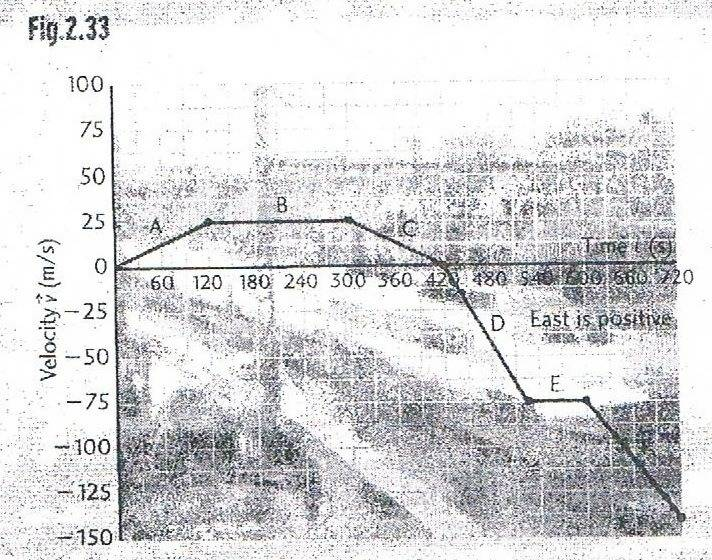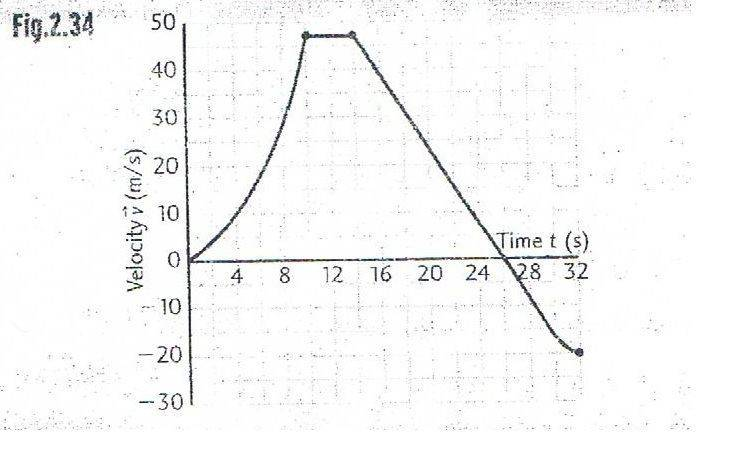# Difference between Average Speed & Average Velocity in Velocity-Time graphs?

I just want to know what is the difference on how to find Average velocity and Average speed when I try to find it in Velocity-Time graphs

Also how do you find distance traveled and displacement in these velocity-time graphs.

Please use fairly simple formulas cause I am in highschool and haven't learned calculus and other physics equations.

I know displacement is something to do with finding area.

PeterO
Homework Helper
I just want to know what is the difference on how to find Average velocity and Average speed when I try to find it in Velocity-Time graphs

Also how do you find distance traveled and displacement in these velocity-time graphs.

Please use fairly simple formulas cause I am in highschool and haven't learned calculus and other physics equations.

I know displacement is something to do with finding area.

Velocity has direction, speed does not. The speedometer in a car measures how fast you are going - but gives no hint on direction.
If we deal with straight line motion, we often label the directions positive and negative, though North, East, South, West, Up and down are other possible names for the directions.

If you positve velocity, you are heading in the positive direction. Mere velocity doesn't say where you are however - as in positive position or negative position.

If you read this response on the same computer as you posted your query, there is every possibility you are now back in the same place. That means your displacement between posting and reading is zero, though you may have travelled a great distance during that time.

The area under a speed time graph gives the distance covered. Note that speed is never negative. It is never positive either. It simply doesn't have direction at all - just magnitude.

If you have a velocity time graph, that graph can dip below the horizontal axis. That is because velocity also has direction, and can be in the positive or negative direction.
When we say the area under a velocity time graph, we actually mean the are between the velocity time graph and the horizontal [time] axis.
Sections above the axis have positive area, areas below the axis have negative area.
When we add all the areas together, there is always a chance that the positive and negative parts may cancel out. This means the object has returned to its original position.

To get distance from a velocity time graph, we find the area between each section and the time axis, ignoring the sign of the area - you just look at the magnitude.

Velocity has direction, speed does not. The speedometer in a car measures how fast you are going - but gives no hint on direction.
If we deal with straight line motion, we often label the directions positive and negative, though North, East, South, West, Up and down are other possible names for the directions.

If you positve velocity, you are heading in the positive direction. Mere velocity doesn't say where you are however - as in positive position or negative position.

If you read this response on the same computer as you posted your query, there is every possibility you are now back in the same place. That means your displacement between posting and reading is zero, though you may have travelled a great distance during that time.

The area under a speed time graph gives the distance covered. Note that speed is never negative. It is never positive either. It simply doesn't have direction at all - just magnitude.

If you have a velocity time graph, that graph can dip below the horizontal axis. That is because velocity also has direction, and can be in the positive or negative direction.
When we say the area under a velocity time graph, we actually mean the are between the velocity time graph and the horizontal [time] axis.
Sections above the axis have positive area, areas below the axis have negative area.
When we add all the areas together, there is always a chance that the positive and negative parts may cancel out. This means the object has returned to its original position.

To get distance from a velocity time graph, we find the area between each section and the time axis, ignoring the sign of the area - you just look at the magnitude.

Most of it. So lets say I had these two graphs:How would I find distance traveled, displacement, speed and velocity from these graphs?

I would appreciate it if you could show your work.

#### Attachments

PeterO
Homework Helper
Most of it. So lets say I had these two graphs:How would I find distance traveled, displacement, speed and velocity from these graphs?

I would appreciate it if you could show your work.

You start by drawing vertical lines from each of the corners of the graph - where you have drawn dots/plotted points.

In the first case this divides the whole thing into a series of triangles and rectangles, which are easy to find the area of.

In Part 1 Sections A,B & C are above the time axis, so represent positive areas for displacement.
Sections D, E & F are below the axis, so represent negative areas for displacement.

Lets pretend the areas under A,B&C was 200, and the Areas fro section D,E&F was -300

That would represent a total displacement of -300, but a distance covered of 500

Since the time was 720 seconds, that would mean average velocity = -100/720 while the average speed would be 500/720

Similar working for part 2 except you have to estimate some areas as the bounding line in some cases is a curve.
If you know the equation of the curve, you could use integration but in physics we usually use either a "counting the squares" technique, or approximate to triangles/rectangles.

hey just wanted to ask a question related to this ...suppose the entire graph is above the horizontal axis so the distance and the displacement will be the same??

i short yes

u can also think like this....

suppose a body moving with velocity v in right direction
Considering the velocity in right direction to be +ve and plotting graph, u can say that when velocity becomes 0 at any instant, i means that the body has stopped moving right.

Again as velocity becomes -ve we can say that body is moving left

now for displacement if u consider right direction to be +ve u must consider left direction to be -ve...

so u can find the area under v-t graph (the area enclosed above +ve axis would give displacement in right direction and area under the +ve x axis would give displacement in left direction)

now add the displacements(with proper signs) to get net final displacement from initial point

now speed dosent consider direction so when body starts moving left, graph would still go up)

here sum of areas of graphs will give us the distance traveled

if the entire graph is above the horizontal axis so the distance and the displacement will be the same as there is no displacement along the opposite direction

NOTE:velocity dosent have to be along left and right it can be up and down or some other any way

thnku :)

one more question...i am given a stair case shaped velocity time graphy above the x-axis with decreasing velocity and i am asked to find instantaneous velocity...should draw tangent at any corner of the stair case and find the slope or what shoud i do i am confused!

if u are given a velocity time graph then u should be able to find instantaneous velocity
by locating the velocity co ordinate for the corresponding time co ordinate

u mean if i have points (1,3) (2,2) (3,1) (4,0) then instantaneous velocity at time 1 would be 3 ??

if it is velocity time graph with time in x axis, then i think yes

instantaneous velocity means velocity at any instant of time

PeterO
Homework Helper
if it is velocity time graph with time in x axis, then i think yes

instantaneous velocity means velocity at any instant of time

I agree with this last post.

If you are considering tangents, you are thinking of ways to get the instantaneous velocity from a position-time graph.